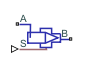# Needle Valve (IL)

Needle valve in an isothermal liquid system

• Library:
• Simscape / Fluids / Isothermal Liquid / Valves & Orifices / Flow Control Valves

•## Description

The Needle Valve (IL) block models flow reductions by a needle valve. The valve comprises a conical needle and a round, sharp-edged seat. The needle opens or closes according to the displacement signal at port S. A positive signal retracts the needle and opens the valve.

### Opening Area

Needle Valve SchematicNeedle Valve Top ViewThe opening area of the valve is calculated as:

`${A}_{open}=\pi h\mathrm{sin}\left(\frac{\theta }{2}\right)\left[{d}_{0}-\frac{h}{2}\mathrm{sin}\left(\theta \right)\right]+{A}_{leak},$`

where:

• h is the vertical distance between the outer edge of the needle and the seat, as indicated in the schematic above.

• θ is the seat cone angle, which always matches the .

• d0 is the Seat orifice diameter.

• Aleak is the Leakage area.

The opening area is bounded by the maximum displacement hmax:

`${h}_{\mathrm{max}}=\frac{{d}_{0}\left[1-\sqrt{1-\mathrm{cos}\left(\frac{\theta }{2}\right)}\right]}{\mathrm{sin}\left(\theta \right)}.$`

For any needle displacement larger than hmax, Aopen is the sum of the maximum orifice area and the Leakage area:

`${A}_{open}=\frac{\pi }{4}{d}_{0}^{2}+{A}_{leak}.$`

For any combination of the signal at port S and the needle offset that is less than 0, the minimum valve area is the Leakage area.

If the parameter is nonzero, the block smoothly saturates the displacement between `0` and hmax. For more information, see Numerical Smoothing.

### Mass Flow Rate Equation

Mass is conserved through the valve:

`${\stackrel{˙}{m}}_{A}+{\stackrel{˙}{m}}_{B}=0.$`

The mass flow rate through the valve is calculated as:

`$\stackrel{˙}{m}=\frac{{C}_{d}{A}_{valve}\sqrt{2\overline{\rho }}}{\sqrt{P{R}_{loss}\left(1-{\left(\frac{{A}_{valve}}{{A}_{port}}\right)}^{2}\right)}}\frac{\Delta p}{{\left[\Delta {p}^{2}+\Delta {p}_{crit}^{2}\right]}^{1/4}},$`

where:

• Cd is the Discharge coefficient.

• Avalve is the instantaneous valve open area.

• Aport is the Cross-sectional area at ports A and B.

• $\overline{\rho }$ is the average fluid density.

• Δp is the valve pressure difference pApB.

The critical pressure difference, Δpcrit, is the pressure differential associated with the Critical Reynolds number, Recrit, the flow regime transition point between laminar and turbulent flow:

`$\Delta {p}_{crit}=\frac{\pi \overline{\rho }}{8{A}_{valve}}{\left(\frac{\nu {\mathrm{Re}}_{crit}}{{C}_{d}}\right)}^{2}.$`

Pressure loss describes the reduction of pressure in the valve due to a decrease in area. PRloss is calculated as:

`$P{R}_{loss}=\frac{\sqrt{1-{\left(\frac{{A}_{valve}}{{A}_{port}}\right)}^{2}\left(1-{C}_{d}^{2}\right)}-{C}_{d}\frac{{A}_{valve}}{{A}_{port}}}{\sqrt{1-{\left(\frac{{A}_{valve}}{{A}_{port}}\right)}^{2}\left(1-{C}_{d}^{2}\right)}+{C}_{d}\frac{{A}_{valve}}{{A}_{port}}}.$`

Pressure recovery describes the positive pressure change in the valve due to an increase in area. If you do not wish to capture this increase in pressure, set Pressure recovery to `Off`. In this case, PRloss is 1.

## Ports

### Conserving

expand all

Liquid entry or exit port to the valve.

Liquid entry or exit port to the valve.

### Input

expand all

Control member displacement in m, specified as a physical signal. A positive signal retracts the needle and opens the valve.

## Parameters

expand all

Diameter of the seat orifice.

Control member geometry angle. Refer to the Needle Valve Schematic in the valve description.

Needle offset when the valve is closed. A positive, nonzero value indicates a partially open valve. A negative, nonzero value indicates an overlapped valve that remains closed for an initial displacement set by the physical signal at port .

Sum of all gaps when the valve is in the fully closed position. Any area smaller than this value is saturated to the specified leakage area. This contributes to numerical stability by maintaining continuity in the flow.

Areas at the entry and exit ports A and B, which are used in the pressure-flow rate equation that determines the mass flow rate through the valve.

Correction factor accounting for discharge losses in theoretical flows.

Upper Reynolds number limit for laminar flow through the orifice.

Continuous smoothing factor that introduces a layer of gradual change to the flow response when the valve is in near-open or near-closed positions. Set this value to a nonzero value less than one to increase the stability of your simulation in these regimes.

Whether to account for pressure increase when fluid flows from a region of smaller cross-sectional area to a region of larger cross-sectional area.

## Version History

Introduced in R2020a